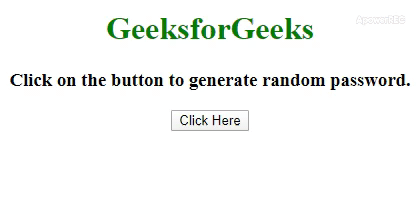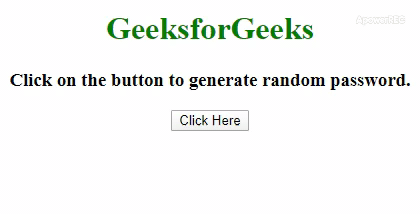# How to Generate a Random Password using JavaScript ?

• Difficulty Level : Medium
• Last Updated : 25 Apr, 2022

The task is to generate a random password that may consist of alphabets, numbers, and special characters. This can be achieved in various ways in this article we will discuss the most popular two methods which are discussed below to solve the problem.

Approach 1: Make a string consist of Alphabets(lowercase and uppercase), Numbers and Special Characters. the we will use Math.random() and Math.floor() method to generate a number in between 0 and l-1(where l is length of string). To get the character of the string of a particular index we can use .charAt() method. This will keep concatenating the random character from the string until the password of the desired length is obtained.

• Example: This example implements the above approach.

 ` ` `<``html``> ` ` `  `<``head``> ` `    ``<``title``> ` `        ``Generate a Random Password ` `        ``using JavaScript ` `    `` ` ` ` ` `  `<``body` `style``=``"text-align:center;"``> ` `     `  `    ``<``h1` `style``=``"color: green"``>  ` `        ``GeeksforGeeks  ` `    `` ` `     `  `    ``<``h3``> ` `        ``Click on the button to ` `        ``generate random password. ` `    `` ` `     `  `    ``<``button` `onclick``=``"gfg_Run()"``> ` `        ``Click Here ` `    `` ` `    ``<``br``> ` `     `  `    ``<``div``> ` `        ``<``p` `id``=``"geeks"``> ` `    `` ` `     `  `    ``<``script``> ` `        ``var el_down = document.getElementById("geeks"); ` ` `  `        ``/* Function to generate combination of password */ ` `        ``function generateP() { ` `            ``var pass = ''; ` `            ``var str = 'ABCDEFGHIJKLMNOPQRSTUVWXYZ' +  ` `                    ``'abcdefghijklmnopqrstuvwxyz0123456789@#\$'; ` `             `  `            ``for (let i = 1; i <= 8; i++) { ` `                ``var char = Math.floor(Math.random() ` `                            ``* str.length + 1); ` `                 `  `                ``pass += str.charAt(char) ` `            ``} ` `             `  `            ``return pass; ` `        ``} ` ` `  `        ``function gfg_Run() { ` `            ``el_down.innerHTML = generateP(); ` `        ``} ` `    `` ` ` ` ` `  ` `

• Output:Approach 2: In this approach we will use Math.random() method to generate a number in between 0 and 1 then convert it to base36(which will consist of 0-9 and a-z in lowercase letters) using .toString() method. To remove the leading zero and decimal point .slice() method will be used and Math.random().toString(36).slice(2) to generate the password. For uppercase letters use the same method with .uppercase() method in concatenation with the previous method.

• Example: This example implements the above approach.

 `  ` `<``html``>  ` ` `  `<``head``>  ` `    ``<``title``>  ` `        ``Generate a Random Password ` `        ``using JavaScript ` `    `` ` `  ` ` `  `<``body` `style` `= ``"text-align:center;"``>  ` `     `  `    ``<``h1` `style` `= ``"color: green"``>  ` `        ``GeeksforGeeks  ` `    `` ` `     `  `    ``<``h3``> ` `        ``Click on the button to ` `        ``generate random password. ` `    `` ` `     `  `    ``<``button` `onclick` `= ``"gfg_Run()"``>  ` `        ``Click Here ` `    `` ` `     `  `    ``<``p` `id` `= ``"geeks"``> ` `     `  `    ``<``script``> ` `        ``var el_down = document.getElementById("geeks"); ` `         `  `        ``function gfg_Run() { ` `            ``el_down.innerHTML =  ` `                ``Math.random().toString(36).slice(2) +  ` `                ``Math.random().toString(36) ` `                    ``.toUpperCase().slice(2); ` `            ``}  ` `    ``  ` `  ` ` `  `       `

• Output:My Personal Notes arrow_drop_up
Recommended Articles
Page :